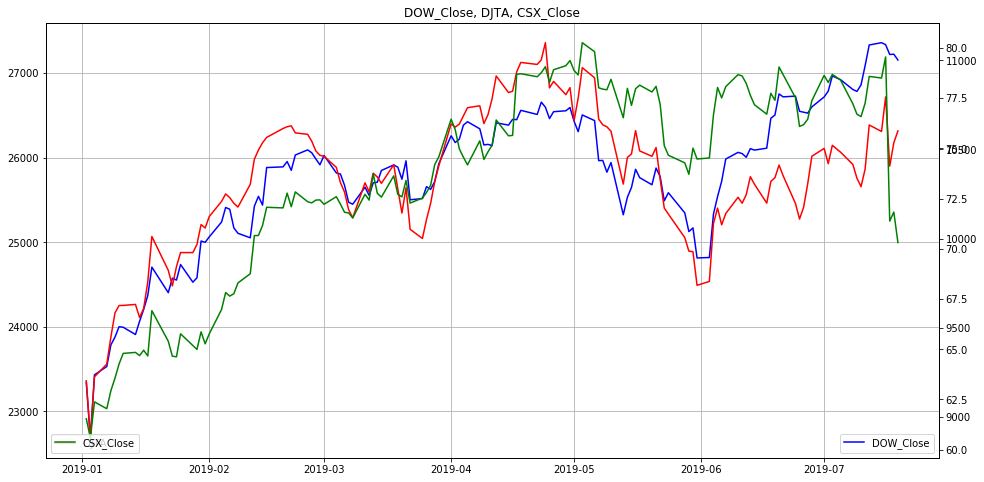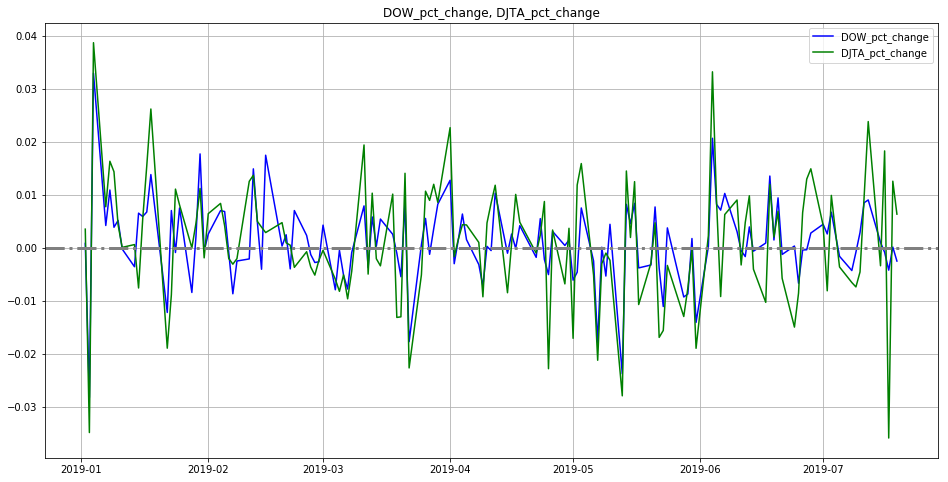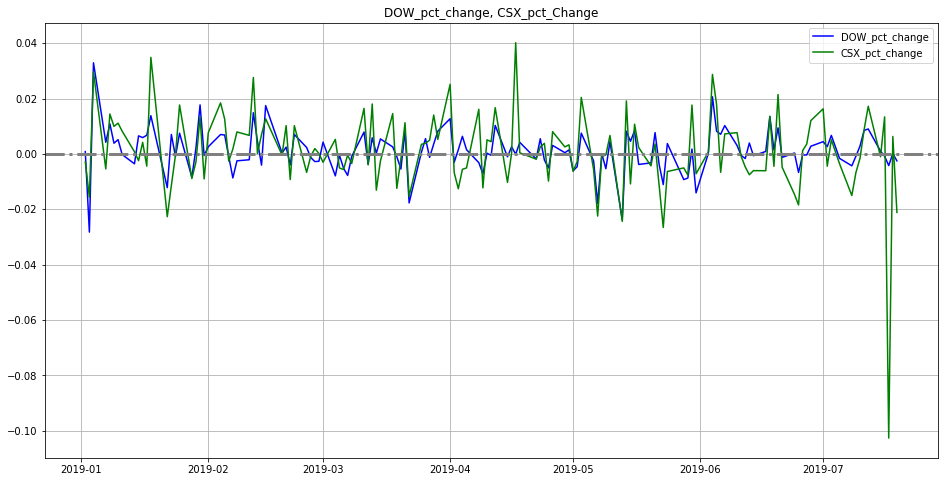### My New Udemy Class: Fundamental Market Analysis with PythonFundamental Market Analysis with Python - Find Your Own Answers On What Is Going on in the Financial Markets

### Hot off the Press!My New Book: "The Little Book of Fundamental Analysis: Hands-On Market Analysis with Python" is Out!

### Grow Your Web Brand, Visibility & Traffic Organically5 Years of amunategui.github.Io and the Lessons I Learned from Growing My Online Community from the Ground Up

### Introduction

Dow Jones Transportation Average Diverting, Trouble Ahead? What about the rumblings in the market that transportation companies are slowing down? It's all related to the Dow theory that states that both industrials and transportation need to be moving upwards for a healthy market. Let's find out for ourselves with raw data and Python!

### Code

ViralML-Hands-Dow-Theory-Transportation-Average
In :
from IPython.display import Image
Image(filename='viralml-book.png')

Out:# Fundamental and Technical Indicators - Hands-On Market Analysis¶

Companion book: "The Little Book of Fundamental Market Indicators":

https://amzn.to/2DERG3d

More at:

https://www.viralml.com/

## Charles Dow and the Dow Jones Transportation and Industrial Indexes¶

https://www.investopedia.com/terms/d/dowtheory.asp

The Dow theory is a theory that says the market is in an upward trend if one of its averages (industrial or transportation) advances above a previous important h igh and is accompanied or followed by a similar advance in the other average.

## Data¶

Dow Jones Industrial Average (^DJI) https://finance.yahoo.com/quote/%5EDJI

Dow Jones Transportation Average (DJTA) https://fred.stlouisfed.org/series/DJTA

### CSX Corporation (CSX)¶

CSX Corporation is an American holding company focused on rail transportation and real estate in North America, among other industries

https://finance.yahoo.com/quote/CSX

In :
%matplotlib inline
import matplotlib
import matplotlib.pyplot as plt
import io, base64, os, json, re
import pandas as pd
import numpy as np
import datetime
import warnings
warnings.filterwarnings('ignore')

In :
path_to_market_data = '/Users/manuel/Documents/financial-research/market-data/2019-07-20/'

# create an MACD indicators
def MACD(df, feature, fast=9, medium=12, long=26):
# WARNING - Feed data in ascending order only (i.e. first row should be your oldest print)

tmp_df = df.copy()

tmp_df['EXP1'] = tmp_df[feature].ewm(span=medium, adjust=False).mean()
tmp_df['EXP2'] = tmp_df[feature].ewm(span=long, adjust=False).mean()
tmp_df['MACD'] = tmp_df['EXP1']-tmp_df['EXP2']
tmp_df['EXP3'] = tmp_df['MACD'].ewm(span=fast, adjust=False).mean()
tmp_df['MACD_Hist'] = tmp_df['MACD'] - tmp_df['EXP3']

return tmp_df


# Data¶

In :
# DJI
dow_df = pd.read_csv(path_to_market_data + '^DJI.csv')
dow_df['Date'] = pd.to_datetime(dow_df['Date'])

dow_df = dow_df[['Date','Adj Close']]
dow_df.columns = ['Date', 'DOW_Close']
print(np.min(dow_df['Date'] ),np.max(dow_df['Date'] ))
dow_df = dow_df.sort_values('Date', ascending=True)
dow_df['DOW_pct_change'] = dow_df['DOW_Close'].pct_change()

dow_df.tail()

1985-01-29 00:00:00 2019-07-19 00:00:00

Out:
Date DOW_Close DOW_pct_change
8684 2019-07-15 27359.160156 0.000993
8685 2019-07-16 27335.630859 -0.000860
8686 2019-07-17 27219.849609 -0.004236
8687 2019-07-18 27222.970703 0.000115
8688 2019-07-19 27154.199219 -0.002526
In :
# DJTA
djt_df = pd.read_csv(path_to_market_data + 'DJTA.csv')
djt_df['Date'] = pd.to_datetime(djt_df['DATE'])

djt_df = djt_df[['Date','DJTA']]
djt_df.columns = ['Date', 'DJTA']

# remove invalid data and cast to float
djt_df = djt_df[djt_df['DJTA'] != '.']
djt_df['DJTA'] = djt_df['DJTA'].astype('float64')
print(np.min(djt_df['Date'] ),np.max(djt_df['Date'] ))
djt_df = djt_df.sort_values('Date', ascending=True)
djt_df['DJTA_pct_change'] = djt_df['DJTA'].pct_change()

djt_df.tail()

2009-07-20 00:00:00 2019-07-19 00:00:00

Out:
Date DJTA DJTA_pct_change
2605 2019-07-15 10601.03 -0.003411
2606 2019-07-16 10794.59 0.018259
2607 2019-07-17 10406.93 -0.035912
2608 2019-07-18 10537.51 0.012547
2609 2019-07-19 10604.20 0.006329
In :
# CSX
csx_df = pd.read_csv(path_to_market_data + 'CSX.csv')
csx_df['Date'] = pd.to_datetime(csx_df['Date'])

csx_df = csx_df[['Date','Adj Close']]
csx_df.columns = ['Date', 'CSX_Close']
print(np.min(csx_df['Date'] ),np.max(csx_df['Date'] ))
csx_df = csx_df.sort_values('Date', ascending=True)
csx_df['CSX_pct_change'] = csx_df['CSX_Close'].pct_change()

csx_df.tail()

1980-11-03 00:00:00 2019-07-19 00:00:00

Out:
Date CSX_Close CSX_pct_change
9755 2019-07-15 78.500000 -0.001018
9756 2019-07-16 79.550003 0.013376
9757 2019-07-17 71.379997 -0.102703
9758 2019-07-18 71.830002 0.006304
9759 2019-07-19 70.309998 -0.021161

# Let's Plot it All¶

In :
cut_off_date = '2019-01-01'

dow_df_tmp = dow_df.copy()
djt_df_tmp = djt_df.copy()
csx_df_tmp = csx_df.copy()

dow_df_tmp = dow_df_tmp[dow_df_tmp['Date'] >= cut_off_date]
djt_df_tmp = djt_df_tmp[djt_df_tmp['Date'] >= cut_off_date]
csx_df_tmp = csx_df_tmp[csx_df_tmp['Date'] >= cut_off_date]

In :
# join both datasets together
fig, ax = plt.subplots(figsize=(16, 8))
plt.plot(dow_df_tmp['Date'], dow_df_tmp['DOW_Close'] , color='blue', label='DOW_Close')
plt.title('DOW_Close, DJTA, CSX_Close')
plt.legend(loc='lower right')
plt.grid()

# Get new axis
ax2 = ax.twinx()
plt.plot(djt_df_tmp['Date'], djt_df_tmp['DJTA'] , color='red', label='DJTA')
plt.legend(loc='lower left')

# Get new axis
ax3 = ax.twinx()
plt.plot(csx_df_tmp['Date'], csx_df_tmp['CSX_Close'] , color='green', label='CSX_Close')
plt.legend(loc='lower left')

plt.show()## As Percentage Change¶

In :
# join both datasets together
fig, ax = plt.subplots(figsize=(16, 8))
plt.plot(dow_df_tmp['Date'], dow_df_tmp['DOW_pct_change'] , color='blue', label='DOW_pct_change')
plt.title('DOW_pct_change, DJTA_pct_change')
plt.plot(djt_df_tmp['Date'], djt_df_tmp['DJTA_pct_change'] , color='green', label='DJTA_pct_change')
plt.axhline(0, color='gray', linewidth=3, linestyle='-.' )
plt.legend()
plt.grid()
plt.show()In :
# join both datasets together
fig, ax = plt.subplots(figsize=(16, 8))
plt.plot(dow_df_tmp['Date'], dow_df_tmp['DOW_pct_change'] , color='blue', label='DOW_pct_change')
plt.title('DOW_pct_change, CSX_pct_Change')
plt.plot(csx_df_tmp['Date'], csx_df_tmp['CSX_pct_change'] , color='green', label='CSX_pct_change')
plt.axhline(0, color='gray', linewidth=3, linestyle='-.' )
plt.legend()
plt.grid()
plt.show()### Show Notes

(pardon typos and formatting -
these are the notes I use to make the videos)

Dow Jones Transportation Average Diverting, Trouble Ahead? What about the rumblings in the market that transportation companies are slowing down? It's all related to the Dow theory that states that both industrials and transportation need to be moving upwards for a healthy market. Let's find out for ourselves with raw data and Python!